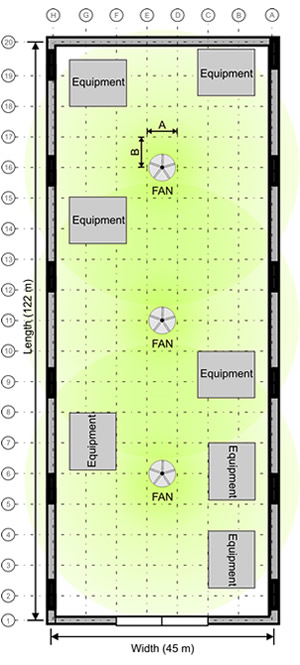### Rep Our Products

Are you interested in representing CaptiveAire and selling our products?
Please fill out the following form, and we will contact you soon.

## How to Calculate the number of Fans1. Calculate the inside floor area of your building:
Length x Width = m² 122 x 45 = 5,490 m² Length x Width = ft² 400 x 148 = 59,200 ft²
2. Locate the structural columns or walls and measure the allowable distance. This will provide you with the max. fan diameter possible that can be installed in the space provide. See "A" and "B" for this example. We will say that both "A" and "B" are 6.7 m (22 ft) apart.
3. Obstructions such as floor equipment, walls or areas set out for storage must be calculated as these objects have direct impact on air circulation. Calculate the m² (ft²) areas of the equipment and figure the percentage of unobstructed space. For this example we will say the equipment in the space takes up 30% of the floor area leaving 70% unobstructed space.
4. In this example, we can install 6.1 m (20 ft) fans due to the 6.7 m (22 ft) column spacing. As per the catalogue specifications, the maximum effective diameter is 61.0 m (200 ft) per 6.1 m (20 ft) fan.
We then calculate the m² (ft²) area that each fan will cover: Radius = Ø / 2 Radius = 61.0 / 2 (Radius = 200 / 2) Radius = 30.5 m (Radius = 100 ft) Area = πr² Area = 3.14 x 30.5² (Area = 3.14 x 100²) Area per fan is 2,921 m². (Area per fan is 31,400 ft².)
5. We then must calculate the number of fans required for the building size. We take the area per fan 2,921 m² (31,400 ft²) multiplied by the percentage of unobstructed area (70%).
2,921 m² x .7 = 2,045 (31,400 ft² x .7 = 21,980)

We then divide this number into the total area of the building.

5,490 m² / 2,045 = 2.70 (59,200 ft² / 21,980 = 2.70)

We require three 6.1 m (20 ft) fans realizing that some overlap of coverage will result.